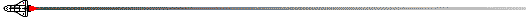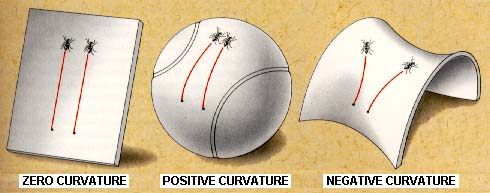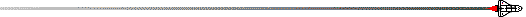## Question:

What is the shape of the universe?One of the most profound insights of General Relativity was the conclusion that mass caused space to curve, and objects travelling in that curved space have their paths deflected, exactly as if a force had acted on them. If space itself is curved, there are three general possibilities for the geometry of the universe. Each of these possibilites is tied to the amount of mass (and thus to the total strength of gravitation) in the universe, and each implies a different past and future for the universe.

First, let's look at shapes and curvatures for a two-dimensional surface. Mathematicians distinguish 3 qualitatively different classes of curvature, as illustrated in the following image:The flat surface at the left is said to have zero curvature, the spherical surface is said to have positive curvature, and the saddle-shaped surface is said to have negative curvature.

The preceding is not too difficult to visualize, but General Relativity asserts that space itself (not just an object in space) can be curved, and furthermore, the space of General Relativity has 3 space-like dimensions and one time dimension, not just two as in our example above. This IS difficult to visualize! Nevertheless, it can be described mathematically by the same methods that mathematicians use to describe the 2-dimensional surfaces. So what do the three types of curvature - zero, positive, and negative -mean to the universe?

• If space has negative curvature, there is insufficient mass to cause the expansion of the universe to stop. In such a case, the universe has no bounds, and will expand forever. This is called an open universe.

• If space has no curvature (i.e, it is flat), there is exactly enough mass to cause the expansion to stop, but only after an infinite amount of time. Thus, the universe has no bounds and will also expand forever, but with the rate of expansion gradually approaching zero after an infinite amount of time. This is termed a flat universe or a Euclidian universe (because the usual geometry of non-curved surfaces that we learn in high school is called Euclidian geometry).

• If space has positive curvature, there is more than enough mass to stop the present expansion of the universe. The universe in this case is not infinite, but it has no end (just as the area on the surface of a sphere is not infinite but there is no point on the sphere that could be called the "end"). The expansion will eventually stop and turn into a contraction. Thus, at some point in the future the galaxies will stop receding from each other and begin approaching each other as the universe collapses on itself. This is called a closed universe.

The geometry of the universe is often expressed in terms of the "density parameter", which is defined as the ratio of the actual density of the universe to the critical density that would be required to cause the expansion to stop. Thus, if the universe is flat (contains just the amount of mass to close it) the density parameter is exactly 1, if the universe is open with negative curvature the density parameter lies between 0 and 1, and if the universe is closed with positive curvature the density parameter is greater than 1.

The density parameter determined from various methods such as calculating the number of baryons created in the big bang, counting stars in galaxies, and observing the dynamics of galaxies both near and far. With some rather large uncertainties, all methods point to the universe being open (i.e. the density parameter is less than one). But we need to remember that it is unlikely that we have detected all of the matter in the universe yet.

The current theoretical belief (because it is predicted by the theory of cosmic inflation) is that the universe is flat, with exactly the amount of mass required to stop the expansion (the corresponding average critical density that would just stop the is called the closure density). Recent observations (such as the BOOMERANG and MAXIMA cosmic microwave background radiation results, and various supernova observations) imply that the expansion of the universe is accelerating. If so, this strongly suggests that the universe is geometrically "flat".

In reality, determining the value of the density parameter and thus the ultimate fate of the universe remains one of the major unsolved problems in modern cosmology. The recently (June 30, 2001) launched MAP mission will be able to measure the value definitively within the next 5 years.The StarChild site is a service of the High Energy Astrophysics Science Archive Research Center (HEASARC), Dr. Alan Smale (Director), within the Astrophysics Science Division (ASD) at NASA/ GSFC.

StarChild Authors: The StarChild Team
StarChild Graphics & Music: Acknowledgments
StarChild Project Leader: Dr. Laura A. Whitlock
Curator: J.D. Myers
Responsible NASA Official: Phil Newman